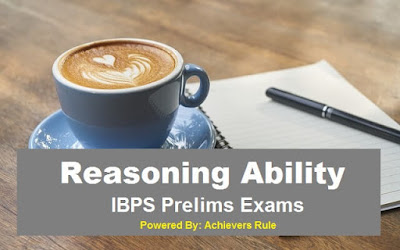# IBPS PO Clerk Reasoning Parctice Set

##Reasoning Ability: Practice Set for IBPS Exam

Q.1. In a row of 22 students, when Milan was shifted towards left by five places he became 12th from the left end. What was his position from the right end of the row?
a). 6th
b). 10th
c). 5th
d). 7th
e). None of this.

Q2. In a class Sagar ranks 18th from the top and 13th from the bottom. If there are six girl students in the class, how many boy students are there?
a). 25
b). 24
c). 26
d). Cannot be determined
e). None of this.

Q3. How many 6’s are there in the following number series which are exactly divisible by its immediate preceding as well as immediately succeeding numbers?

3     6     4     2    6     3      8      6      3      6      4      2      6       3        8      6    9    6    3    6   4   6   2

a). None
b). One
c). Two
d). Three
e). None of this.

Q.4. In a certain code PROVISION is written as NOISIVORP. How is RECTANGLE written in that code?
a). ELGNATREC
b). ELGNATCER
c). GLENATCER
d). ELGTANCER
e). None of these.

Q5.If the letters in the word STENOGRAPHY are arranged in alphabetical order, which letter would be in the 7th position from the right end?
a). H
b). O
c). P
d). R
e). None of these.

Directions (Q.6 – Q.10) Study the following information to answer the given questions:
• Seven persons A, B, C, D, E, F and G came and left a party follows.
• The person who came last did not leave last but the person who came first also left first.
• G came before A, E and C, and E came before D, B and C.
• F left before A and D, and C left after E and D, B left after D and before E.
• F came before E but after A, D came before C.
• A left before B and D.

Q.6. who was fifth to came?
a). E
b). B
c). D
d). cannot be determined
e). None of this.

Q.7 which of the following is the correct sequence of coming starting from the one who came first?
a). GAFEBDC
b). GAFEBCD
c). GAFEDCB
d). cannot be determined
e). None of these.

Q8. Who came in the end?
a). B
b). C
c). Either D or B
d). cannot be determined
e). None of these.

Q.9 Which of the following is the correct sequence of leaving, starting form the person who left first.
a). GFDABEC
c). FGDABEC
d). cannot be determined
e). None of these

Q.10. Who left first?
a). F
b). D
c). C
d). cannot be determined
e). None of this.

Directions (Q.11 – Q.15.) Study the following information to answer the given questions:
• Six students P, Q, R, S, T and U are in different branches of engineering, i.e., civil, electrical, mechanical, chemical, metallurgy and electronics but not necessary in the same order.
• Each of them is a resident of a different city i.e. Calcutta, Mumbai, Chennai, Delhi, Bangalore and Hyderabad. R is the resident of Delhi but he is not in chemical or electrical, T, who is in mechanical, is not the resident of Mumbai or Hyderabad. Q is from Calcutta and he is in electrical. The student from Chennai is in electronics and S is from Mumbai. P is in Metallurgy.

Q.11. R is study which subject?
a).electronics.
b). mechanical.
c). metallurgy.
e). None of this.

Q.12 Which student is from Bangalore?
a). P
b). Q
c). S
d).T or P
e). None of this.

Q.13 which of the following is not correct combination of students and subject?
a). Q-electrical
b). U-electronics
c). P-metallurgy
d). S-civil
e). None of this.

Q.14 Which students is from Chennai?
a). R
b). U
c). S
d). T
e). None of this

Q.15. P is from which city?
a). Chennai
b). Calcutta
e). None of this
SOLUTION

Ans.1. –a)
Milan position from the right end =22 – (12 + 5) + 1 =6th.

Ans.2 – b)
Total number of students = 18 + 13 – 1 = 30
Total number of boys = 30 – 6 = 24

Ans.3 – c).

Ans.4 – b)
Letters of the word are written in reverse order in the code.

Ans. 5 – e)
The letters in the word STENOGRAPHY can be arranged in alphabetical order as AEGHNOPRSTY. N is he seventh from the right.

Ans. 6 – 10.
LEAVING SEQUENCE: GFDABEC
COMING SEQUENCE: GAFEDCB

Ans. 11– 15
 Student State Branch R Delhi Civil T Bangalore Mechanical Q Calcutta Electrical U Chennai Electronics S Mumbai Chemical P Hyderabad Metallurgy